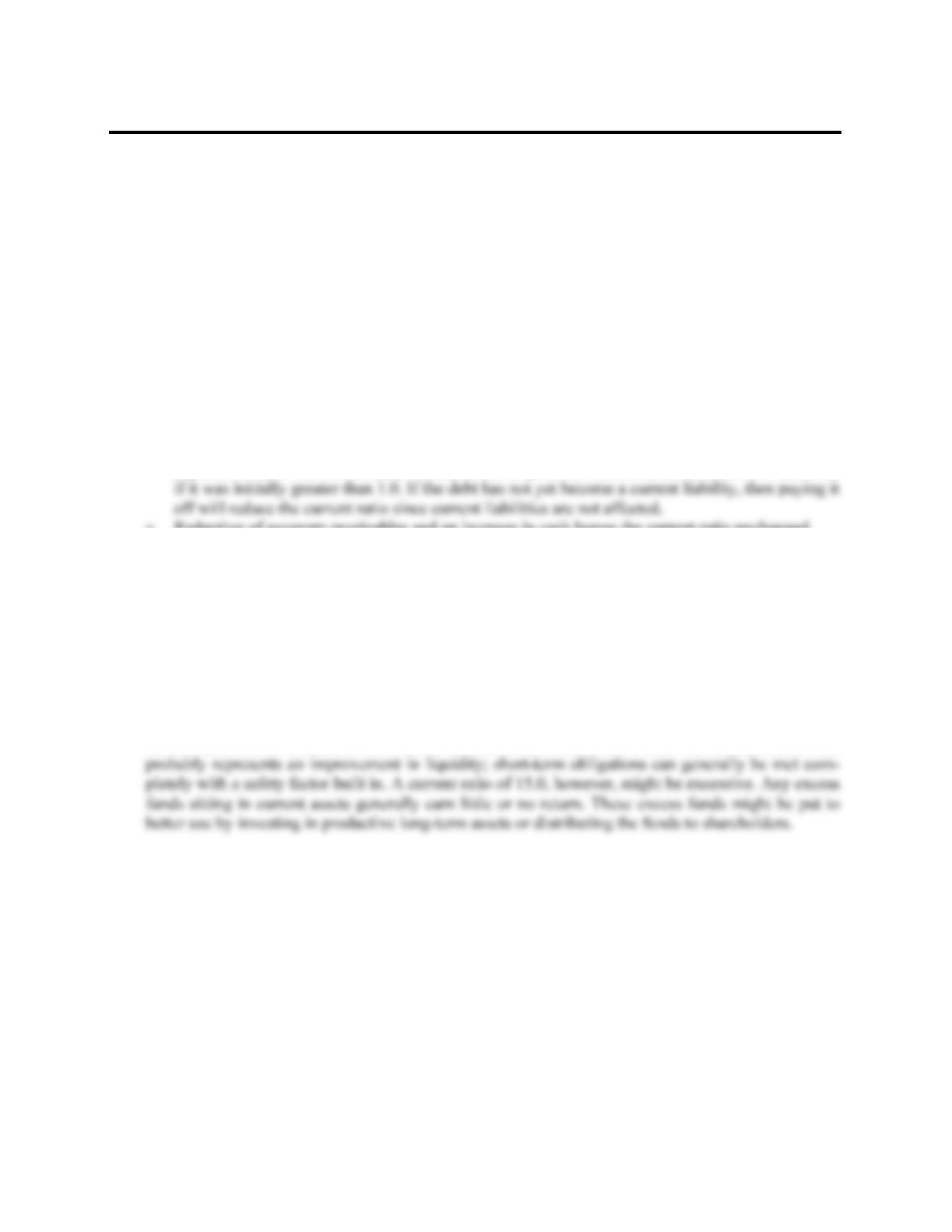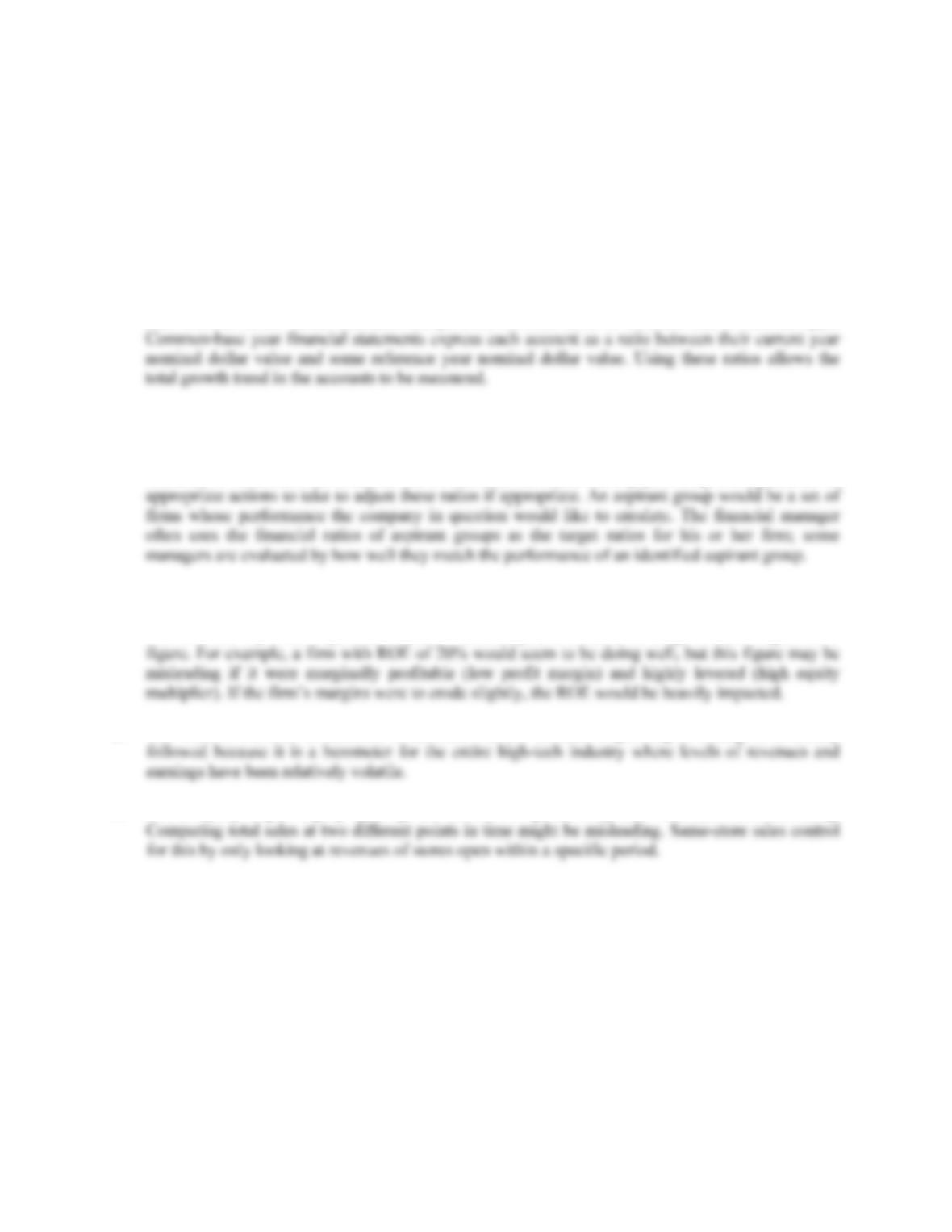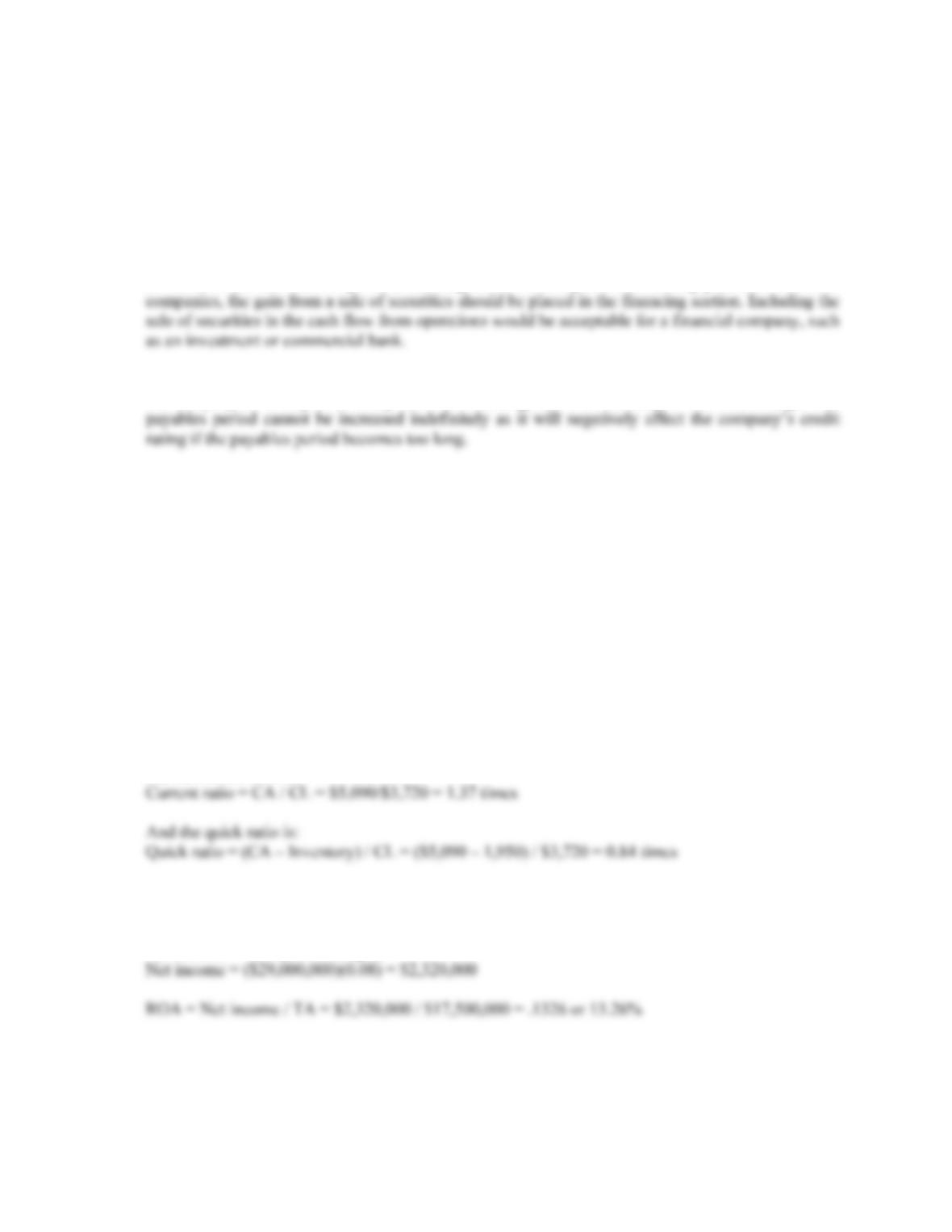Type
Quiz
Book Title
Fundamentals of Corporate Finance Standard Edition 9th Edition
ISBN 13
978-0073382395

### 978-0073382395 Chapter 3 Concepts Review and Critical Thinking Questions

April 3, 2019CHAPTER 3
WORKING WITH FINANCIAL
STATEMENTS
Answers to Concepts Review and Critical Thinking Questions
1. a. If inventory is purchased with cash, then there is no change in the current ratio. If inventory is
purchased on credit, then there is a decrease in the current ratio if it was initially greater than 1.0.
b. Reducing accounts payable with cash increases the current ratio if it was initially greater than 1.0.
c. Reducing short-term debt with cash increases the current ratio if it was initially greater than 1.0.
d. As long-term debt approaches maturity, the principal repayment and the remaining interest
expense become current liabilities. Thus, if debt is paid off with cash, the current ratio increases
e. Reduction of accounts receivables and an increase in cash leaves the current ratio unchanged.
f. Inventory sold at cost reduces inventory and raises cash, so the current ratio is unchanged.
g. Inventory sold for a profit raises cash in excess of the inventory recorded at cost, so the current
ratio increases.
2. The firm has increased inventory relative to other current assets; therefore, assuming current liability
levels remain unchanged, liquidity has potentially decreased.
3. A current ratio of 0.50 means that the firm has twice as much in current liabilities as it does in
current assets; the firm potentially has poor liquidity. If pressed by its short-term creditors and
suppliers for immediate payment, the firm might have a difficult time meeting its obligations. A
current ratio of 1.50 means the firm has 50% more current assets than it does current liabilities. This
4. a. Quick ratio provides a measure of the short-term liquidity of the firm, after removing the effects
of inventory, generally the least liquid of the firm’s current assets.
b. Cash ratio represents the ability of the firm to completely pay off its current liabilities with its
most liquid asset (cash).
c. Total asset turnover measures how much in sales is generated by each dollar of firm assets.
d. Equity multiplier represents the degree of leverage for an equity investor of the firm; it measures
the dollar worth of firm assets each equity dollar has a claim to.
e. Long-term debt ratio measures the percentage of total firm capitalization funded by long-term
debt.B-16 SOLUTIONS
f. Times interest earned ratio provides a relative measure of how well the firm’s operating earnings
can cover current interest obligations.
g. Profit margin is the accounting measure of bottom-line profit per dollar of sales.
h. Return on assets is a measure of bottom-line profit per dollar of total assets.
i. Return on equity is a measure of bottom-line profit per dollar of equity.
j. Price-earnings ratio reflects how much value per share the market places on a dollar of
accounting earnings for a firm.
5. Common size financial statements express all balance sheet accounts as a percentage of total assets
and all income statement accounts as a percentage of total sales. Using these percentage values rather
than nominal dollar values facilitates comparisons between firms of different size or business type.
6. Peer group analysis involves comparing the financial ratios and operating performance of a
particular firm to a set of peer group firms in the same industry or line of business. Comparing a firm
to its peers allows the financial manager to evaluate whether some aspects of the firm’s operations,
finances, or investment activities are out of line with the norm, thereby providing some guidance on
7. Return on equity is probably the most important accounting ratio that measures the bottom-line
performance of the firm with respect to the equity shareholders. The Du Pont identity emphasizes the
role of a firm’s profitability, asset utilization efficiency, and financial leverage in achieving an ROE
8. The book-to-bill ratio is intended to measure whether demand is growing or falling. It is closely
9. If a company is growing by opening new stores, then presumably total revenues would be rising.
10. a. For an electric utility such as Con Ed, expressing costs on a per kilowatt hour basis would be a
way to compare costs with other utilities of different sizes.
b. For a retailer such as Sears, expressing sales on a per square foot basis would be useful in
comparing revenue production against other retailers.
c. For an airline such as Southwest, expressing costs on a per passenger mile basis allows for
comparisons with other airlines by examining how much it costs to fly one passenger one
mile.CHAPTER 3 B-17
d. For an on-line service provider such as AOL, using a per call basis for costs would allow for
comparisons with smaller services. A per subscriber basis would also make sense.
e. For a hospital such as Holy Cross, revenues and costs expressed on a per bed basis would be
useful.
f. For a college textbook publisher such as McGraw-Hill/Irwin, the leading publisher of finance
textbooks for the college market, the obvious standardization would be per book sold.
11. Reporting the sale of Treasury securities as cash flow from operations is an accounting “trick”, and
as such, should constitute a possible red flag about the companies accounting practices. For most
12. Increasing the payables period increases the cash flow from operations. This could be beneficial for
the company as it may be a cheap form of financing, but it is basically a one time change. The
Solutions to Questions and Problems
NOTE: All end of chapter problems were solved using a spreadsheet. Many problems require multiple
steps. Due to space and readability constraints, when these intermediate steps are included in this
solutions manual, rounding may appear to have occurred. However, the final answer for each problem is
found without rounding during any step in the problem.
Basic
1. Using the formula for NWC, we get:
NWC = CA – CL
CA = CL + NWC = \$3,720 + 1,370 = \$5,090
So, the current ratio is:
2. We need to find net income first. So:
Profit margin = Net income / Sales
Net income = Sales(Profit margin)